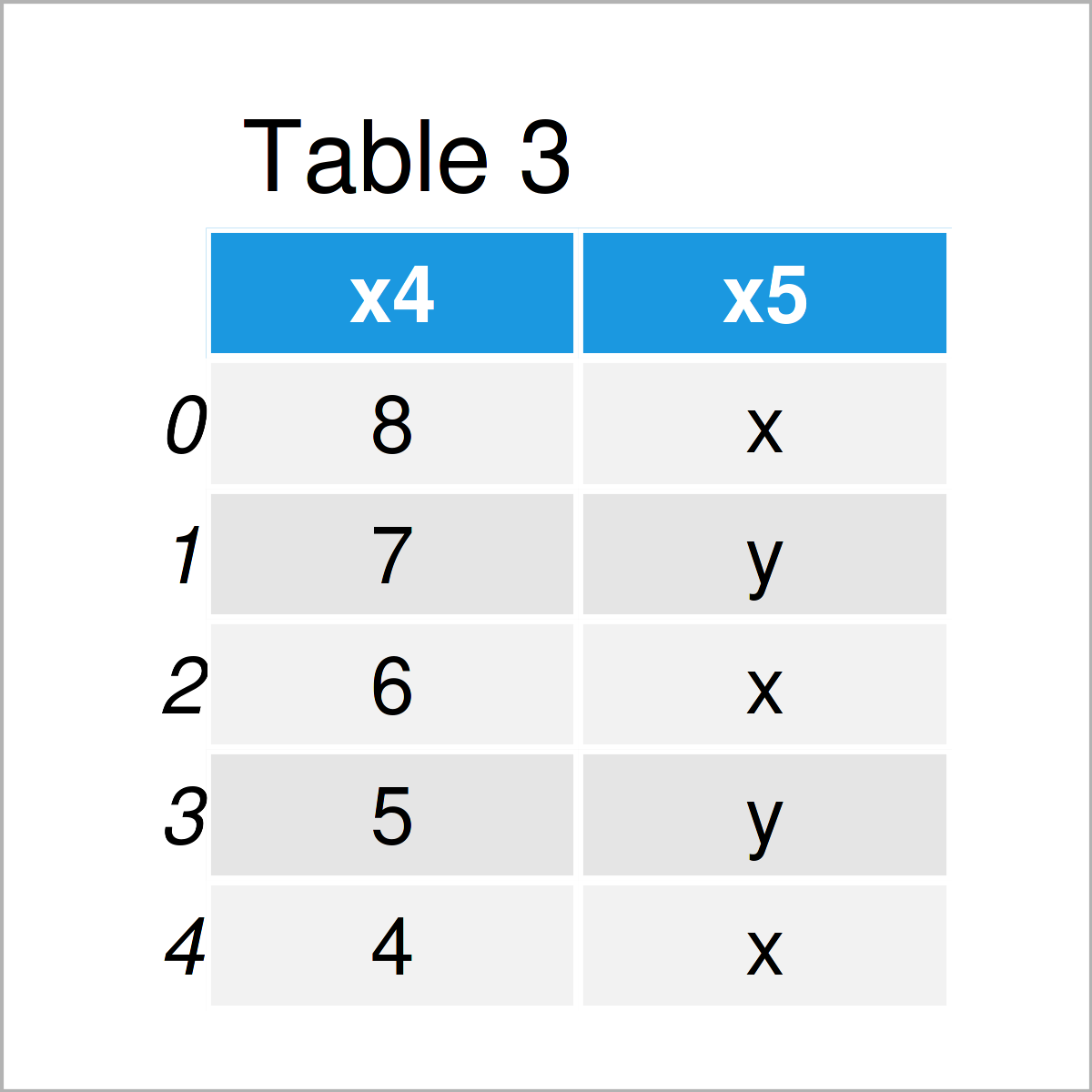# Extract First & Last N Columns from pandas DataFrame in Python (2 Examples)

In this tutorial you’ll learn how to return the first and last N columns from a pandas DataFrame in the Python programming language.

The post is structured as follows:

Let’s dig in!

## Example Data & Add-On Libraries

We first have to import the pandas library, in case we want to use the functions that are contained in the library:

`import pandas as pd                         # Load pandas library`

Furthermore, consider the following example data:

```data = pd.DataFrame({'x1':range(10, 15),    # Create pandas DataFrame
'x2':range(0, 5),
'x3':['a', 'b', 'c', 'd', 'e'],
'x4':range(8, 3, - 1),
'x5':['x', 'y', 'x', 'y', 'x']})
print(data)                                 # Print pandas DataFrame```Have a look at the table that has been returned after executing the previous Python programming code. It shows that our example DataFrame contains five rows and five columns.

## Example 1: Get First N Columns from pandas DataFrame

In Example 1, I’ll demonstrate how to select the first N variables of a pandas DataFrame in Python.

To accomplish this, we can use the iloc attribute as shown below:

```data_first_n = data.iloc[:, :3]             # Select first columns
print(data_first_n)                         # Print first columns```The output of the previous Python programming syntax is shown in Table 2: We have created a new pandas DataFrame subset containing only the first three columns of our input DataFrame.

## Example 2: Get Last N Columns from pandas DataFrame

This example demonstrates how to return only the last N columns from a pandas data set.

Similar to Example 1, we can use the iloc attribute for this. Note that we have to specify the column index from which we want to extract the columns in front of the : instead of after the : sign (as we did in Example 1).

```data_last_n = data.iloc[:, 3:]              # Select last columns
print(data_last_n)                          # Print last columns```After executing the previous Python programming code the updated pandas DataFrame shown in Table 3 has been created. As you can see, we have extracted only the last two columns.

## Video & Further Resources

Do you want to learn more about the extraction of the first and last N columns from a pandas DataFrame? Then you may watch the following video on my YouTube channel. In the video, I’m explaining the Python syntax of this article in the Python programming language:

Please accept YouTube cookies to play this video. By accepting you will be accessing content from YouTube, a service provided by an external third party.If you accept this notice, your choice will be saved and the page will refresh.

Furthermore, you might have a look at some of the related tutorials that I have published on this homepage:

This tutorial has illustrated how to extract the first and last N columns from a pandas DataFrame in Python (similar to head and tail for rows). In case you have further questions and/or comments, please let me know in the comments section below.

Subscribe to the Statistics Globe Newsletter